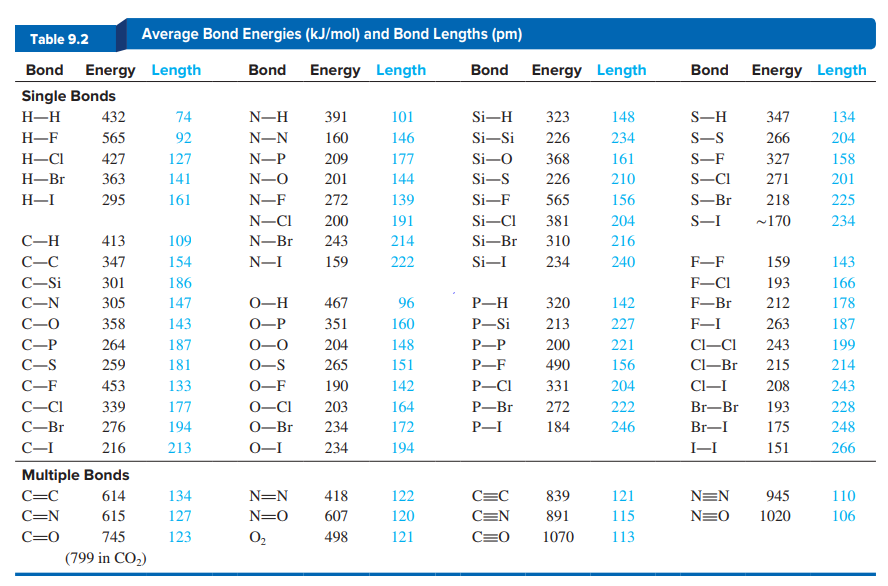# Problem: Using bond lengths in Table 9.2 and assuming ideal bond angles, calculate each of the following distances:(c) Between equatorial F atoms in PF5

###### FREE Expert Solution
83% (106 ratings)
###### Problem Details

Using bond lengths in Table 9.2 and assuming ideal bond angles, calculate each of the following distances:

(c) Between equatorial F atoms in PF5